# High School Physics : Period of SHM

## Example Questions

### Example Question #1 : Period Of Shm

Basic Computational

A spring has a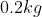mass attached to one, which oscillates with a period of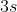. What is the frequency?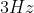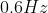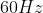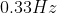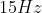Explanation:

The mass has no bearing on the relationship between frequency and period. This relationship is given by the equation: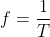Given the period, the frequency will be equal to its reciprocal.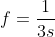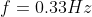### Example Question #2 : Period Of Shm

A spring has a spring constant of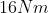.   If a force of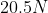is used to stretch out the spring, what is the total displacement of the spring?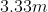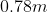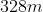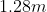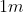Explanation:

For this problem, use Hooke's law: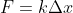In this formula,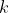is the spring constant,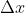is the compression of the spring, and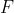is the necessary force. We are given the spring constant and the force, allowing us to solve for the displacement.

Plug in our given values and solve.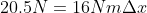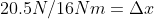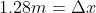Note that both the force and the displacement are positive because the stretching force will pull in the positive direction. If the spring were compressed, the change in distance would have been negative.

### Example Question #3 : Period Of Shm

A spring is attached to a mass, oscillating freely in simple harmonic motion. What change can be made to increase the period of the oscillation?

Take the spring to a planet with a lower acceleration due to gravity

Decrease the mass at the end of the spring

Use a spring with a greater spring constant

Increase the mass at the end of the spring

Lengthen the spring

Increase the mass at the end of the spring

Explanation:

The equation for the period of a spring in simple harmonic motion is: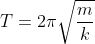In this formula, m is the mass and k is the spring constant. The only two things we can adjust that can change the period, then, are the mass and spring constant. The length of the spring and the acceleration due to gravity are irrelevant.

If we increase the mass we get a larger numerator, which in turns will give us a larger period. If we decrease the mass we get a smaller numerator, which would give us a smaller period. If we use a higher spring constant we get a larger denominator, which also gives us a smaller period.

### Example Question #4 : Period Of Shm

A man swings a bucket back and forth at the end of a rope, creating a pendulum. What factor could be used to increase the period of the pendulum?

Move the pendulum to a lower altitude

Increase the length of the rope

Remove water from the bucket

Use a stronger rope

Increase the length of the rope

Explanation:

The equation for the period of a pendulum is: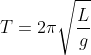Notice that the material of the pendulum and the mass at the end do not enter into the equation at all. The only things that are capable of affecting the period are the length of the pendulum and the acceleration due to gravity.

While changing the altitude of the pendulum will change the period, it will do it only slightly unless you take it miles above the earth's crust. Furthermore, decreasing the altitude of the pendulum will increase the force due to gravity, which will result in a decreased period. The best answer is to increase the length of the rope. This will increase the period of the pendulum.

### Example Question #5 : Period Of Shm

Your grandfather clock’s pendulum has a length of.  If the clock loses half a minute per day, how should you adjust the length of the pendulum?

We should shorten the pendulum by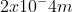We should lengthen the pendulum by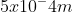We should shorten the pendulum by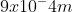We should length the pendulum byWe should lengthen the pendulum byWe should lengthen the pendulum byExplanation:

We also can calculate the total number of seconds in a day.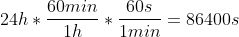There are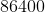seconds in one day.

Therefore we want our clock to swing a certain number of times with a period of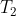to equal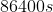.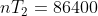We know that our current clock has a certain number of swings with a period of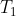to equal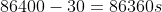So we have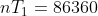We can calculate the current period of the pendulum using the equationWe can set up a ratio of each of these two periods to determine the missing length.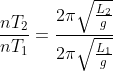Notice that 2, pi and g are all in both the numerator and denominator and therefore fall out of the problem.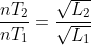We can now solve for our missing piece.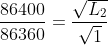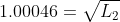Square both sides to get rid of the square root.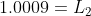We should lengthen the pendulum by### Example Question #6 : Period Of Shm

A mass is placed at the end of a spring. It has a starting velocity of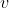and is allowed to oscillate freely. If the mass has a starting velocity of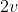, what would the period be?

The period would be 14 as long.

The period would be 4 times as long.

The period would be half as long.

The period would be twice as long.

The period would remain the same.

The period would remain the same.

Explanation:

When it comes to the period of a spring, the velocity of the object has no effect.

The equation is### All High School Physics Resources